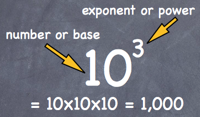## 21 January 2014

### Exponent and Power : Mathematics NCERT / CBSE Notes

• An exponent or power is a mathematical representation that indicates the number of times that a number is multiplied by itself.
• If a number is multiplied by itself m times, then it can be written as: a x a x a x a x a...m times = am
• Here, a is called the base, and m is called the exponent, power or index.
• Numbers raised to the power of two are called square numbers.• Square numbers are also read as two-square, three-square, four-square, five-square, and so on.
• Numbers raised to the power of three are called cube numbers.
• Cube numbers are also read as two-cube, three-cube, four-cube, five-cube, and so on.
• Negative numbers can also be written using exponents.
• If an = b, where a and b are integers and n is a natural number, then an is called the exponential form of b.
• The factors of a product can be expressed as the powers of the prime factors of 100.
• This form of expressing numbers using exponents is called the prime factor product form of exponents.
• Even if we interchange the order of the factors, the value remains the same.
• So a raised to the power of x multiplied by b raised to the power of y, is the same as b raised to the power of y multiplied by a raised to the power of x.
• The value of an exponential number with a negative base raised to the power of an even number is positive.
• If the base of two exponential numbers is the same, then the number with the greater exponent is greater than the number with the smaller exponent.
• A number can be expressed as a decimal number between 1.0 and 10.0, including 1.0, multiplied by a power of 10. Such a form of a number is known as its standard form.

### Laws of Exponents

Multiplication of Powers with the Same Base
When numbers with the same base are multiplied, the power of the product is equal to the sum of the powers of the numbers.

If 'a' is a non-zero integer, and 'm' and 'n' are whole numbers then, am × an = am+n.

Division of Powers with the Same Base
When numbers with the same base are divided, then the power of the quotient is equal to the difference between the powers of the dividend and the divisor.

If 'a' is a non-zero integer, and 'm' and 'n' are whole numbers then, am ÷ an = am-n.

Power of a Power
If 'a' is any non-zero integer, and ‘m’and ‘n’ are whole numbers then, (am)n = amn.

Multiplication of Powers with the Same Exponent
If 'a' is any non-zero integer, and ‘m’ is a whole number then, am × bm = (ab)m.

Division of Powers with the Same Exponent
If a and b are any non-zero integers and m is a whole number then, am ÷ bm = ( a b )m.

Numbers with an exponent of zero
For any non-zero integer a, a0 = 1.

 With our free PDF notes you can get success in any competitive or entrance exams like CTET,  KVS, NET, CAT, MAT, CMAT, SSC, B.ED, IBPS Recruitment, IAS, CSAT, State Civil Services Exams, UPTET, PSTET, HTET & many more. It also provides NCERT solutions, CBSE, NTSE, Olympiad study material, Indian General Knowledge, English, Hindi, Mathematics, Current affairs, Science, S.ST, model test papers, important Questions and Answers asked in CBSE examinations.